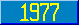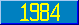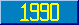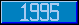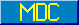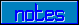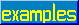## \$ASCII

### 7.1.5.1 \$ASCII

This form produces an integer value as follows:

1. –1 if the value of expr is the empty string.
2. Otherwise, an integer n associated with the leftmost character of the value of expr, such that \$ASCII(\$Char(n)) = n.

This form is similar to \$ASCII(expr) except that it works with the intexprth character of expr instead of the first. Formally, \$ASCII(expr,intexpr) is defined to be \$ASCII(\$Extract(expr,intexpr)).